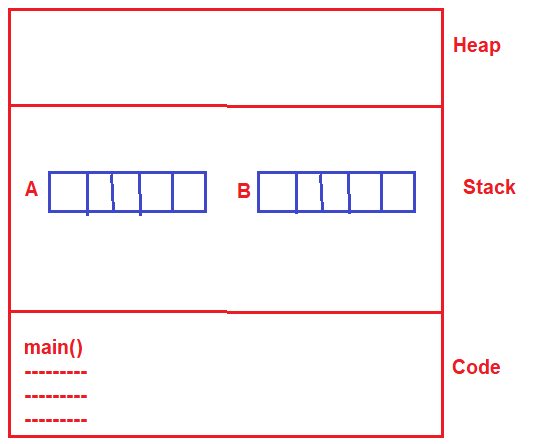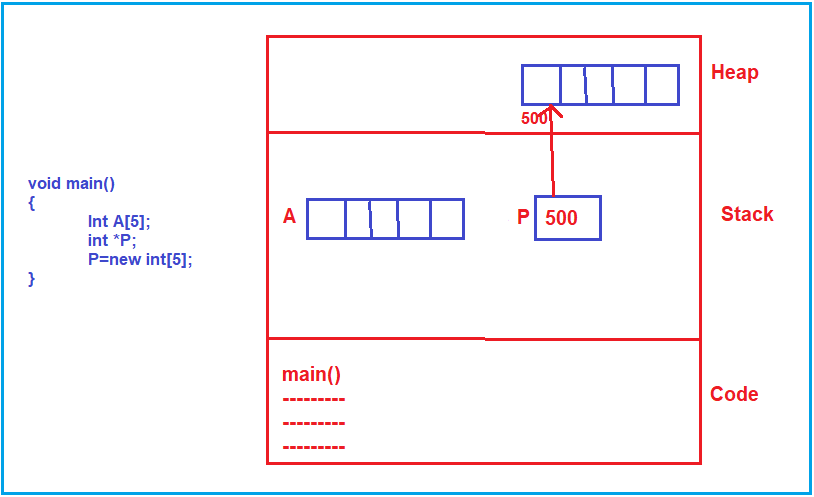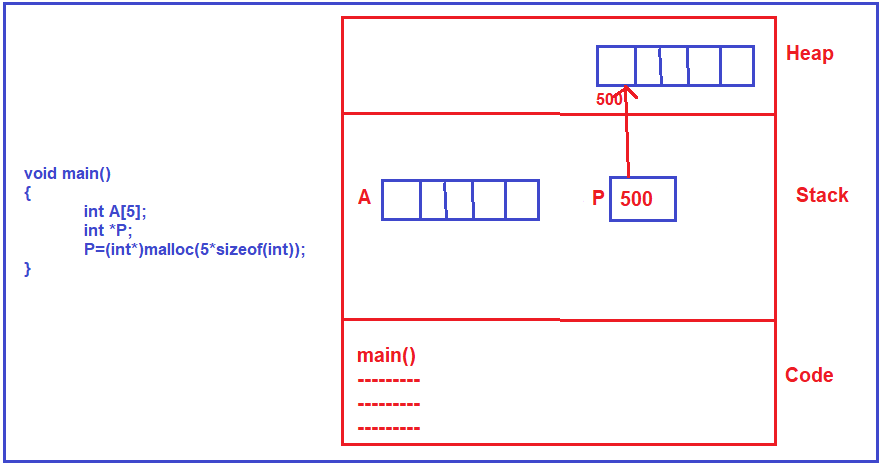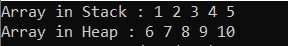# Static vs Dynamic Array in C/C++

## Static vs Dynamic Array in C and C++

In this article, I am going to discuss Static vs Dynamic Array in C and C++ with Examples. Please read our previous article where we discussed Array Declaration and Initialization.

##### Static vs Dynamic Array

Static array means the size of an array is static and dynamic array means the size of an array is dynamic. Once the array is created its size cannot be modified. In our programs when we declare an array, for example, we have a function called main, and inside the main function if we have declared an array i.e. int A; as shown below then an array of size 5 will be created.

void main()
{
int A;
}

Now, the question is where it is created? As it is created inside the main function as a variable (as a vector variable), the memory for this will be created inside the stack. So, an array of size 5 will be created inside the main memory as a part of the activation record of the main function. So, this array will be created inside the stack.

Why we are calling it static? Because the size of this array was decided at compile-time, but the memory will be allocated during the runtime. Memory cannot be allocated at compile time. So, the memory will be allocated during runtime and the size of that memory was decided already at the compilation time.

Definitely, the size of an array has to be decided at compile time in C language. In C language when you are mentioning the size of an array, it must be a constant value, it cannot be a variable.

But in C++, we can create an array of any size at run time. So, at run time the size of an array can be decided and that array will be created inside the stack whereas, in C language, the size has to be decided at compile-time only.

Let us see in C++, suppose we have one variable n and we take the value of n from the keyboard at runtime. Then we can declare an array of size n. So, whatever the inputs come from the keyboard, we can create an array of that particular size, so the size of an array is decided at runtime. For better understanding please have a look at the below code.

void main()
{
int n;
cin>>n;
int B[n];
}

##### Memory Representation:The size of an array can be decided at run time in C++ but in C language it has to be mentioned at compile-time only and that’s the difference.

##### How to create an array inside the heap?

Now, we will learn how to create an array inside the heap. That means an array, whose size and type everything is decided at runtime. The most important thing that you need to remember is for accessing anything from the heap we must have a pointer. So, first of all, we should declare a pointer, and here we declare a pointer with the name P (int *P). Now where this P will get the memory? As P is a variable, so, this will also get memory inside the stack.

Note: In any language when we declare a variable, the memory for that variable will go inside the stack only.

Then the question is when it will be allocated in heap? Let us see how we will use that pointer variable for creating the memory in the heap. The following line of code in C++ will allocate memory for 5 integers inside the heap and the pointer P will be pointing into that address

P=new int;

Suppose assume that the beginning address of that contagious memory in heap is 500, then 500 will be stored into the pointer variable P. Now the program can access the heap by coming on to the pointer and taking the address, and then it can go to that address in heap and access it. So, heap memory cannot be access directly, it has to be accessed indirectly using pointers. For better understanding, please have a look at the following image.Note: One important point that you need to remember is, in C++, when you initialize the variable using the new keyword, then only you will get the memory from heap only, else all the variables get the memory inside the stack.

##### How to create the memory in heap in C?

So, for that, we have to use the built-in malloc function. The malloc function takes the size in bytes i.e. the number of bytes of memory to be allocated inside the help. Here, we want to create 5 integers so we need to mention the size as 5*sizeof(int). The malloc function will allocate just a raw memory or just a block of memory, so, we have to typecast it as an integer pointer i.e., P=(int*)malloc(5*sizeof(int)); For better understanding, please have a look at the following image.When we declare a normal array then it will be created inside the stack and when we wanted an array to be created inside the heap then we must have a pointer and we should allocate the memory either using the new operator (in C++) or using malloc function (in C), then only it will be created inside the heap and the heap memory can be accessed indirectly with the help of pointer.

Now one more important point that you need to remember when we have allocated the memory in heap and after some time during the execution of the program if that memory is no more required then we must delete the memory. If we don’t delete the unused memory then it causes a memory leak problem.

##### How to release or delete the memory?

In C++, we have to say delete []P;

In C language, we have to say free(P);

These are the methods of deallocation in C and C++ language.

##### How to access the array elements from the heap?

We can access the normal array which is created inside the stack like below.
int A;
A= 10;

We can access the array which is created inside the heap using a pointer like below.
int *P;
P=new int;
P =10;

Note: The point that you need to remember is whenever we created an array whether it is created inside the stack or created inside the heap, once the array of some size is created it cannot be resized, its size cannot be changed.

If at all we want to increase the size of an array, it is possible in another way. It is possible only when the array is created in heap, it is not possible if the array is created inside the stack. That we will learn in our upcoming articles.

##### Example: Static vs Dynamic Array

Let us see an example to understand the stack and dynamic array in C. In the following example, array A is created in the stack and another array is created in the heap memory which is referenced by the pointer variable P.

```#include <stdio.h>
#include <stdlib.h>
int main ()
{
int A = { 1, 2, 3, 4, 5 };
int *p;

p = (int *) malloc (5 * sizeof (int));
p = 6;
p = 7;
p = 8;
p = 9;
p = 10;

printf("Array in Stack : ");
for (int i = 0; i < 5; i++)
{
printf ("%d ", A[i]);
}

printf ("\n");
printf("Array in Heap : ");
for (int i = 0; i < 5; i++)
{
printf ("%d ", p[i]);
}
return 0;
}
```

Output:In the next article, I am going to discuss the How to increase the size of an array in C and C++. Here, in this article, I try to explain Static VS Dynamic Array in C and C++ and I hope you enjoy this Static VS Dynamic Array article.